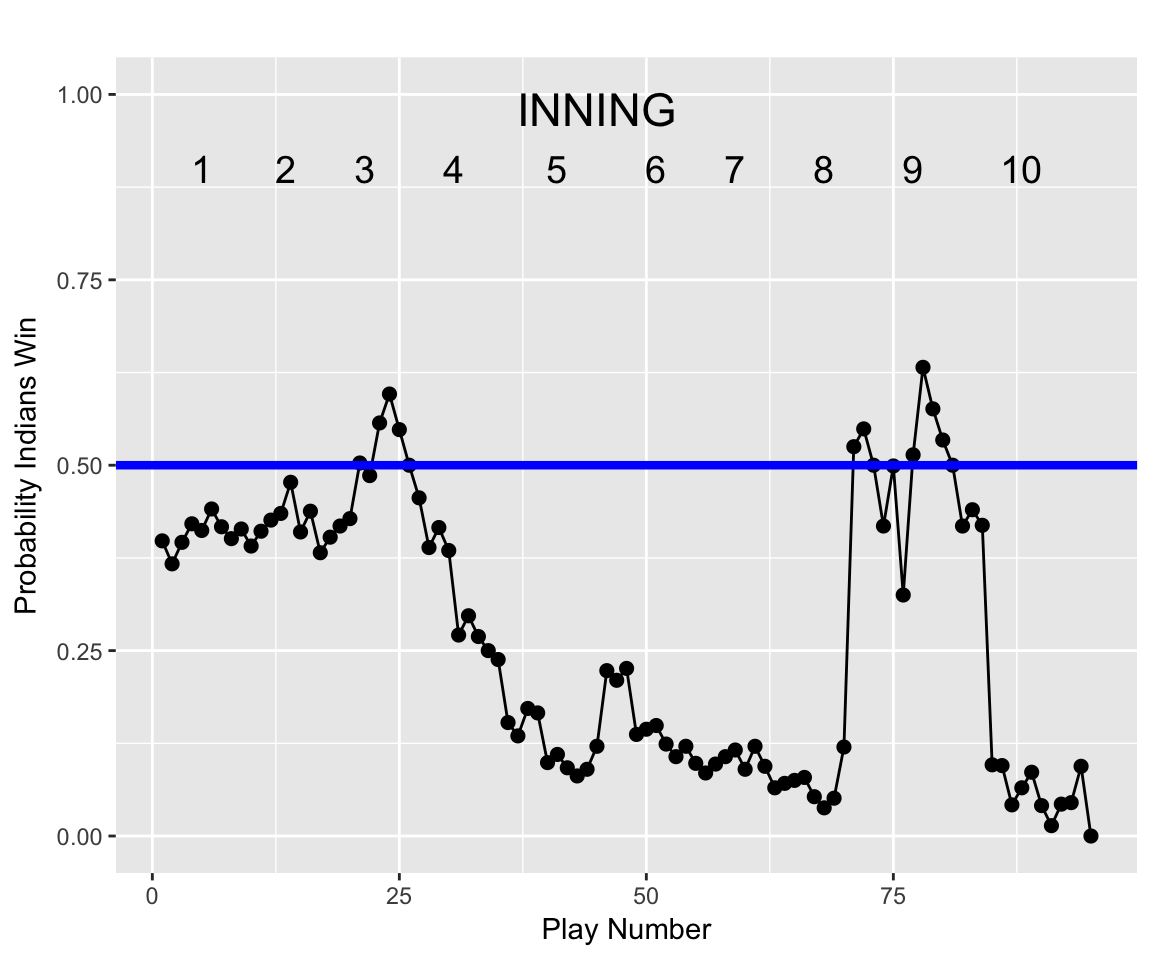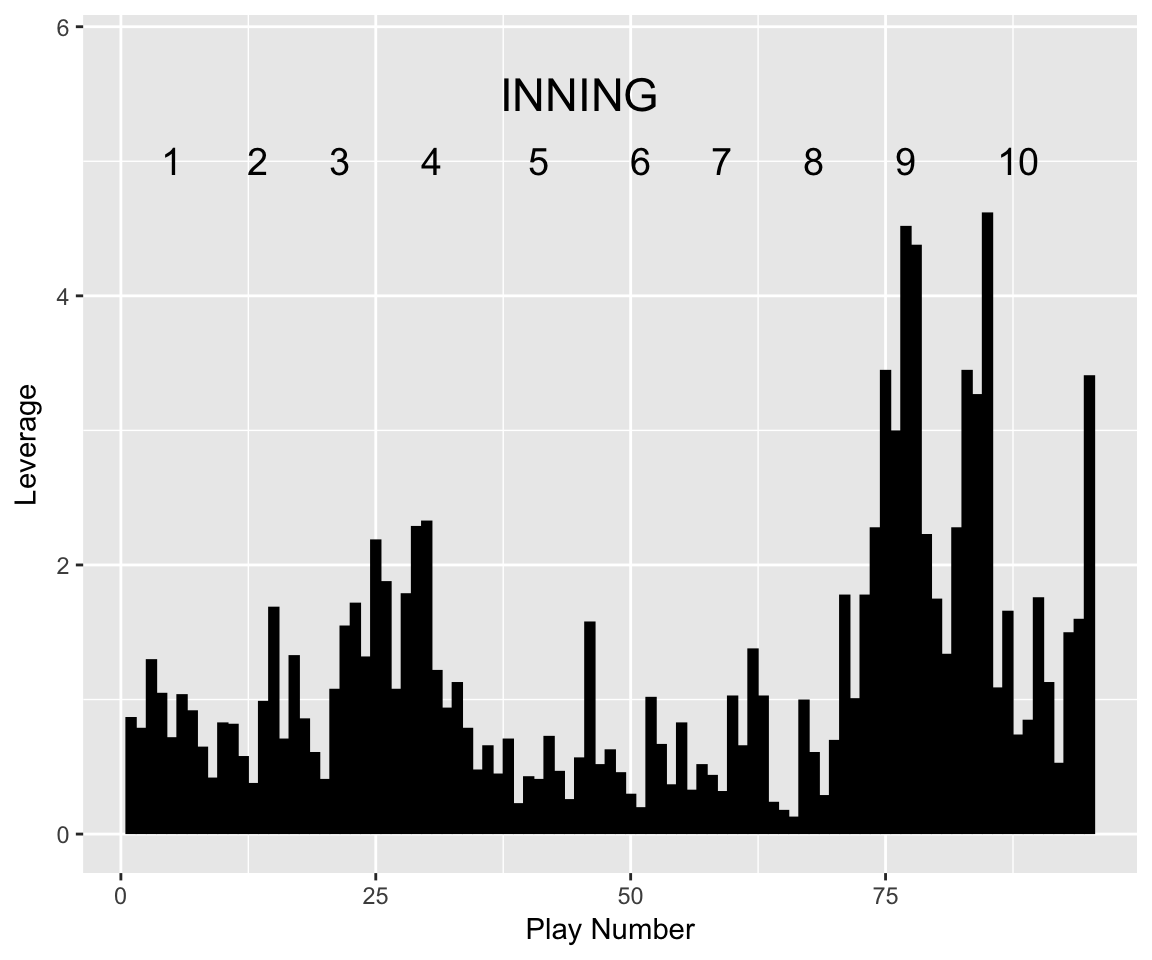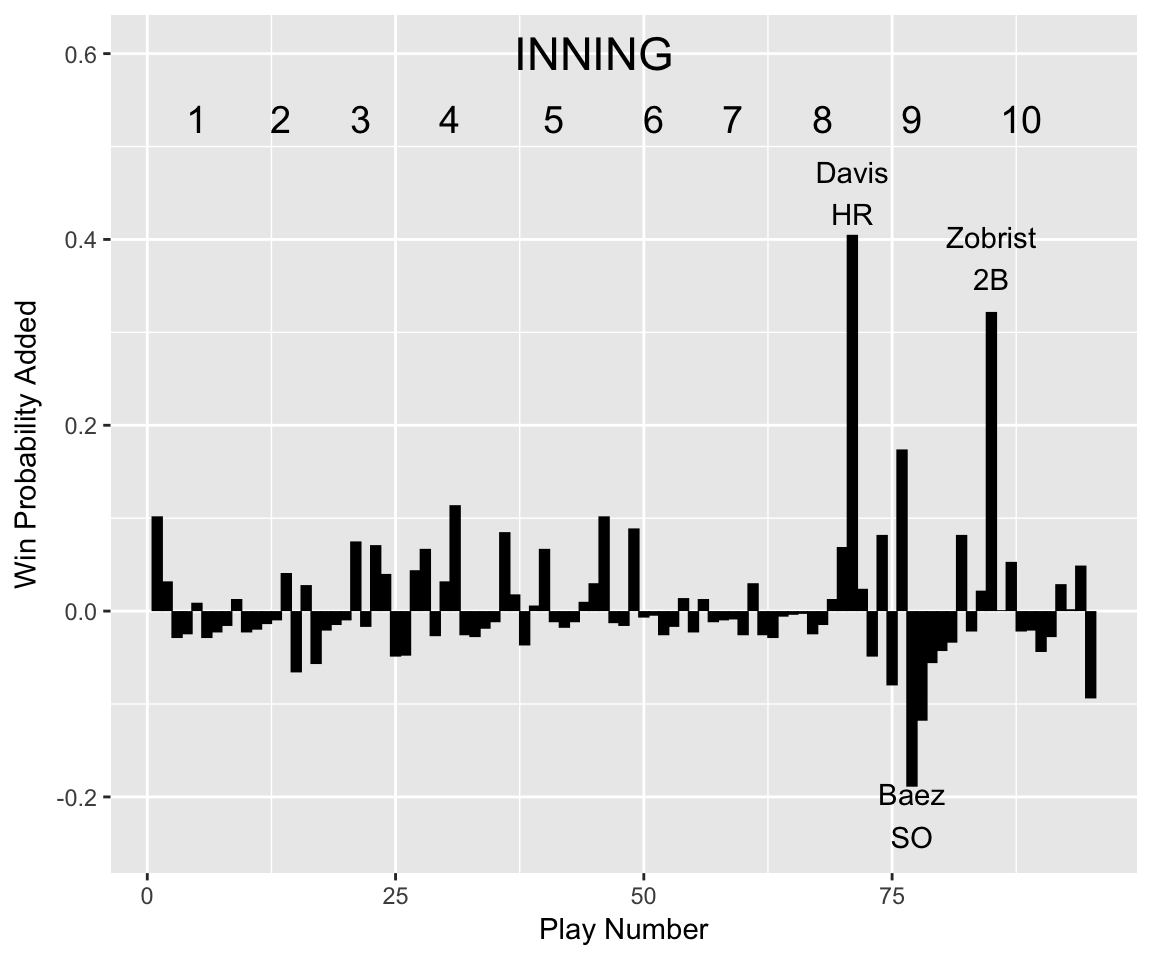library(dplyr)
library(ggplot2)
library(stringr)
library(readr)

#### The Data

The FanGraphs page http://www.fangraphs.com/plays.aspx?date=2016-11-02&team=Indians&dh=0 provides a play log for Game 7 of the 2016 World Series. The table on that page was downloaded and stored in a csv file that is read into R.

d <- read_csv("https://bayesball.github.io/VB/data/WSGame7.csv")
d$Play_Number <- 1:dim(d) d$WE  <- as.numeric(str_replace(d\$WE, "%", ""))
head(d)
## # A tibble: 6 x 13
##    Pitcher      Player  Inn.  Outs  Base Score
##      <chr>       <chr> <int> <int> <chr> <chr>
## 1 C Kluber    D Fowler     1     0   ___   0-1
## 2 C Kluber K Schwarber     1     0   ___   0-1
## 3 C Kluber    K Bryant     1     0   1__   0-1
## 4 C Kluber     A Rizzo     1     1   1__   0-1
## 5 C Kluber K Schwarber     1     2   1__   0-1
## 6 C Kluber   B Zobrist     1     2   _2_   0-1
## # ... with 7 more variables: Play <chr>, LI <dbl>, RE <dbl>, WE <dbl>,
## #   WPA <dbl>, RE24 <dbl>, Play_Number <int>

#### Plot of Win Probabilities of a Game

The WE column of the data frame gives the win probability as a percentage. The below plot graphs the win probability against the Play_Number variable. I add additional text indicating the inning of the game.

ggplot(d, aes(Play_Number, WE / 100)) +
geom_point(size=2) +
geom_line() +
ylim(0, 1) +
ggtitle("") +
ylab("Probability Indians Win") +
geom_hline(yintercept = .50, color="blue", size=1.5) +
annotate("text", x=cumsum(c(0, 10, 7, 9, 9, 12, 8,
8, 10, 8)) +
c(10, 7, 9, 9, 12, 8,
8, 10, 8, 14) / 2,
y=0.90,
label = as.character(1:10), size=5) +
annotate("text", x=45, y=0.98,
label="INNING", size=6) +
xlab("Play Number")#### Plot of Leverages

The variable LI is the leverage of the game situation defined by the score, inning, runners on base and number of outs. This graph plots the leverage values against the play number.

ggplot(d, aes(Play_Number, LI)) +
geom_segment(aes(xend = Play_Number, yend = 0),
size = 2, lineend = "butt") +
xlab("Play Number") +
ylab("Leverage")  +
ylim(0, 5.8) +
annotate("text", x=cumsum(c(0, 10, 7, 9, 9, 12, 8,
8, 10, 8)) +
c(10, 7, 9, 9, 12, 8,
8, 10, 8, 14) / 2,
y=5,
label = as.character(1:10), size=5) +
annotate("text", x=45, y=5.5, label="INNING", size=6)#### Plot of Win Probability Added

The variable WPA provides the change in the win probability for each play. This graph plots WPA against the play number.

ggplot(d, aes(Play_Number, WPA)) +
geom_segment(aes(xend = Play_Number, yend = 0),
size = 2, lineend = "butt") +
xlab("Play Number") +
annotate('text', x=77, y=-0.22, label="Baez\nSO")